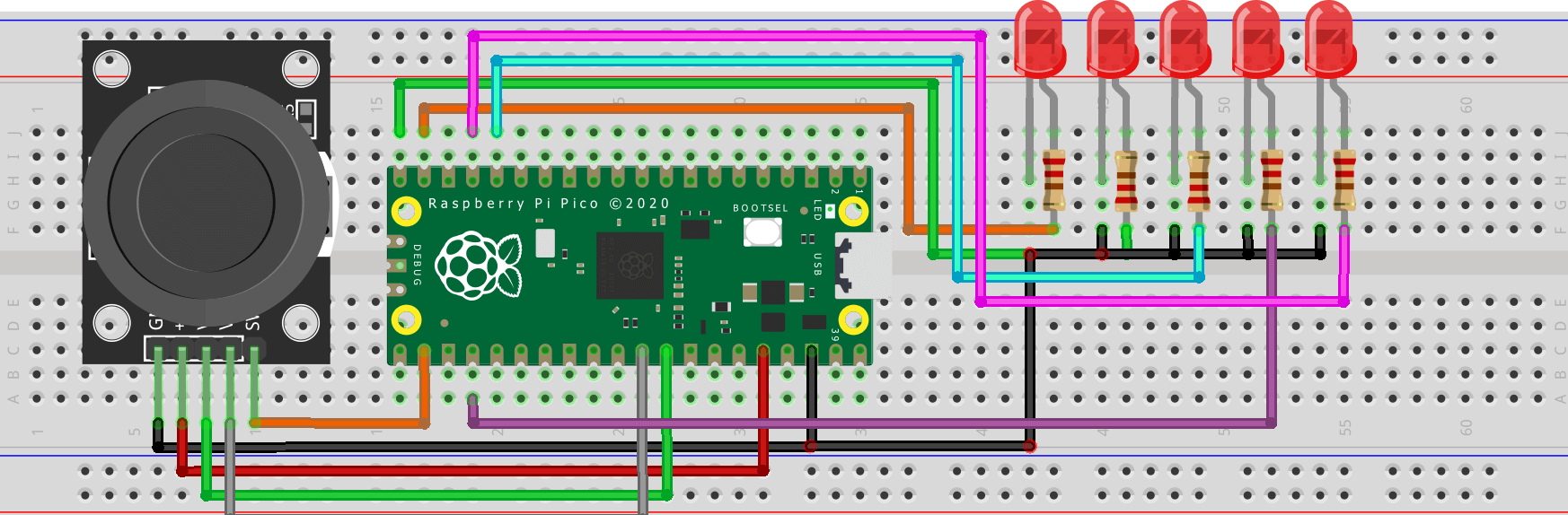Raspberry Pi Pico Projects

# Joystick With Raspberry Pi Pico MicroPython Tutorial

Joystick With Raspberry Pi Pico is a great way to control your next project. You can use the joystick to control anything from a simple LED to a complex robotic arm. The joystick is easy to use and can be interfaced with various electronics. This essay will explore how to interface a Raspberry Pi Pico with a Joystick.  We’ll also look at some example projects that you can use to get started with your own Raspberry Pi Pico and Joystick project.

## Joystick Module

A joystick is an input device that consists of a stick that can be tilted in multiple directions to control an on-screen pointer. A joystick module is used to control a computer or video game character by moving a lever in different directions. It is also used to control other devices such as cameras and robots. Joystick modules come in different sizes and shapes. The module can be used to measure the tilt of a joystick on the X and Y axes.

There are two main types of joysticks:

Analog – Analog joysticks use potentiometers to measure the position of the stick

Digital Digital joysticks use switches to determine the direction the stick is moved.

### Joystick module pinout

This joystick module has five pins which are numbered from left to right for reference:1. GND: Pin connected to ground.
2. +5V: power pin(5v).
3. VRx: potentiometer reading pin for the x’s axis.
4. VRy: potentiometer reading pin for the y’s axis.
5. SW: is an additional pin used for a push button at the bottom.

## Connections Joystick With Raspberry Pi PicoArduino Joystick
`GND` ➡️ `GND`
`5V` ➡️ `+5V`
GP27 (PIN 32) ➡️ `VRx`
`GP25 (PIN 31)` ➡️ `VRy`
`GP17 (PIN 22)` ➡️ `SW`

## Programming – Joystick With Raspberry Pi Pico

Copy all the code and then go to thonny create a new file and paste the below code.

#### Example Code 1

```from machine import Pin, ADC
import utime

button = Pin(17,Pin.IN, Pin.PULL_UP)

while True:
buttonValue = button.value()
buttonStatus = "not pressed"

if buttonValue == 0:
buttonStatus = "pressed"

print("X: " + str(xValue) + ", Y: " + str(yValue) + " -- button value: " + str(buttonValue) + " button status: " + buttonStatus)
utime.sleep(0.2)```

#### Example Code 2

```from machine import Pin, ADC
import utime

button = Pin(17,Pin.IN, Pin.PULL_UP)

led_left = Pin(14, Pin.OUT)
led_middle = Pin(15, Pin.OUT)
led_right = Pin(12, Pin.OUT)
led_up = Pin(18, Pin.OUT)
led_down = Pin(13, Pin.OUT)

while True:
buttonValue = button.value()
xStatus = "middle"
yStatus = "middle"
buttonStatus = "not pressed"

led_left.value(0)
led_down.value(0)
led_up.value(0)
led_right.value(0)
led_middle.value(1)

# Check the x and y Value to determine the status of the joystick
if buttonValue == 0:
buttonStatus = "pressed"
led_middle.value(0)

if xValue <= 600:
xStatus = "left"
led_left.value(1)
led_middle.value(0)

if xValue >= 60000:
xStatus = "right"
led_right.value(1)
led_middle.value(0)

if yValue <= 600:
yStatus = "up"
led_up.value(1)
led_middle.value(0)

if yValue >= 60000:
yStatus = "down"
led_down.value(1)
led_middle.value(0)

print("X: " + xStatus + ", Y: " + yStatus + "  button status: " + buttonStatus)
utime.sleep(0.2)```

### Example 2. Schematic Diagram for Joystick With Raspberry Pi Pico and LED• button = Pin(17,Pin.IN, Pin.PULL_UP)
• led_left = Pin(14, Pin.OUT)
• led_middle = Pin(15, Pin.OUT)
• led_right = Pin(12, Pin.OUT)
• led_up = Pin(18, Pin.OUT)
• led_down = Pin(13, Pin.OUT)